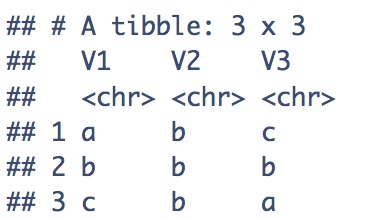[This article was first published on R - Data Science Heroes Blog, and kindly contributed to R-bloggers]. (You can report issue about the content on this page here)
Want to share your content on R-bloggers? click here if you have a blog, or here if you don't.Scenario: we got a table of id-value, and a matrix/tibble that contains the id, and we need the labels.

It may be useful when predicting the Key (or Ids) of in a classification model (like in Keras), and we need the labels as the final output.

There are two interesting things:

• The usage of apply based on column and rows at the same time.
• The creation of an empty tibble and how to fill it (append columns)
``````library(tibble)
# mapping table (id-value)
map_table=tibble(id=c(1,2,3),
value=c("a", "b", "c")
)

map_table
``````
``````## # A tibble: 3 x 2
##      id value
##
## 1     1 a
## 2     2 b
## 3     3 c
``````
``````# given a key, retrun the label
get_label <- function(x)
{
id_flag=map_table\$id==x
res=as.character(map_table[id_flag, 2])
return(res)
}

# the data to get the label
X_data=tibble(v1=c(1,2,3),
v2=c(2,2,2),
v3=c(3,2,1)
)

X_data
``````
``````## # A tibble: 3 x 3
##      v1    v2    v3
##
## 1     1     2     3
## 2     2     2     2
## 3     3     2     1
``````

## Option 1: as matrix

``````mat_res=apply(X_data, 1:2, get_label)

## Checking...
mat_res
``````
``````##      v1  v2  v3
## [1,] "a" "b" "c"
## [2,] "b" "b" "b"
## [3,] "c" "b" "a"
``````

## Option 2: as tibble

``````# creating a 1 column with NAs same length as nrow(X_data)
tib_res=tibble(V1=rep(NA, nrow(X_data)))
for(i in 1:ncol(X_data))
{
vec=X_data[,i]
vec_lbl=sapply(t(vec), get_label) # if X_data is a matrid, no need to transpose with t()
tib_res[,i]=vec_lbl
}

## Checking...
tib_res
``````
``````## # A tibble: 3 x 3
##   V1    V2    V3
##
## 1 a     b     c
## 2 b     b     b
## 3 c     b     a
``````

Option 2, to my surprise, is faster.
I didn’t use the `add_column` because of the need of replacing the first dummy `NA` column.
Other approaches may include dictionaries.

Any improvement in the code is welcome.